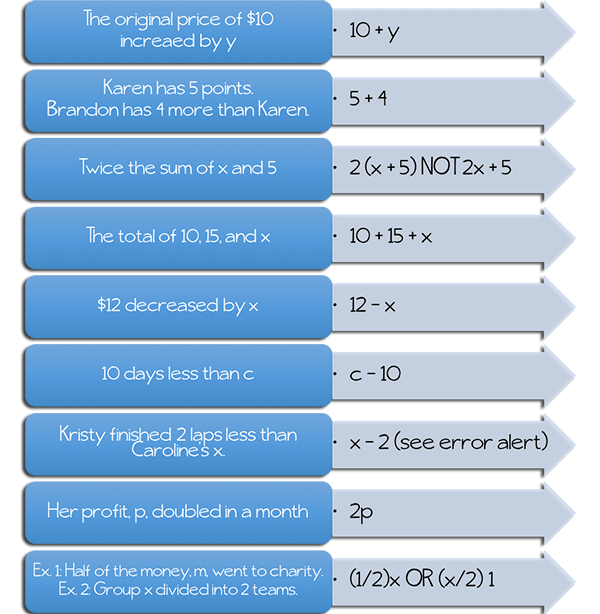# Translate Words to Math

You have learned to translate word problems into mathematical phrases and sentences. In this lesson, you will learn to translate verbal expressions into algebraic expressions, which consist of numbers, variables, and operations. When solving real-world problems, you will need to learn this very important skill. There are key vocabulary words that will help you learn which operation to perform. Below is a list of key words and phrases and how they can be translated into mathematical operations. Take a look at the chart below and become familiar with these words and how they are used for these translations. This chart does not include every situation you might see, but it includes the most common examples.

Operation Verbal Expression Algebraic Expression– a number increased by five

– five added to a number

– the sum of a number and five

– a number plus five

– five more than a number

—————————————–– a number less five

– a number minus five

– a number decreased by five

– the difference of a number and five

– take away five from a number

– five less than a number

——————————————-– five times a number

– five multiplied by a number

– the product of five and a number

———————————————– a number divided by five

– five divided into a number

– the quotient of a number and fiveThe biggest challenge to solving word problems is deciding when to add, subtract, multiply, or divide. When you translate verbal expressions into algebraic expressions, you look for the key vocabulary in each phrase that will provide a clue as to the correct operation to use.To translate real-world problems into algebraic expressions, you must use vocabulary words and key phrases to determine which operation to use.

ERROR ALERT: In subtraction word problems, you cannot always subtract the numbers in the order that they appear in the problem. Sometimes the first number should be subtracted from the last, so it is necessary to read word problems carefully.

Now, take a look below to see more common phrases that are used in word problems and how you can focus on the key vocabulary to help translate the words to math.## Practice

#### Translate the following into mathematical expressions.

1. The quotient of a number and 3

2. Twelve less a number

3. Twelve less than a number

4. The difference of 3 times a number and 5

5. The quotient of 3 and a number, increased by 2

6. The sum of a number and 5, multiplied by 3

7. The product of 3 and a number, increased by 5

8. A number increased by 12

9. Double the number, x

10. Half of the number x

(source)